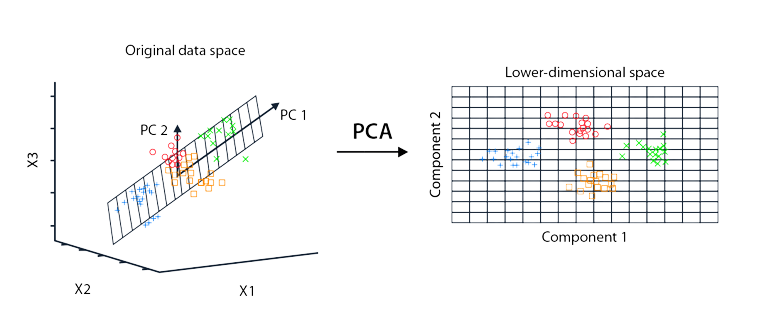Course Content

Principal Component AnalysisIntroduction

Principal component analysis (PCA) - is one of the most well-known data processing algorithms. Sometimes it is used only as a data processing step, after which the obtained data is used in machine learning algorithms. In other cases, PCA is used as the main method for solving practical problems.

PCA - is an unsupervised machine learning method. In order to start using it, it is enough to have a dataset.

What can PCA really do? This is an algorithm that allows you to reduce the dimensionality of the data. Suppose we have data with 125 features and we want to create a machine learning model that will classify this data. If there are more than 500,000 records in the dataset, then training can take too long and take a lot of computing resources. What will be the solution? The use of principal component analysis, which can reduce the dimension of characteristics, for example, to 50.In this course, we only cover the PCA algorithm, but there are many other approaches to data dimensionality reduction.